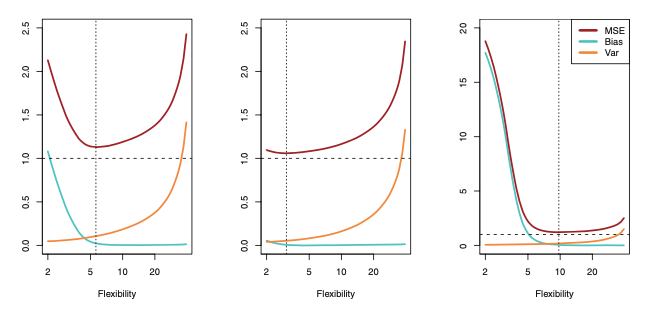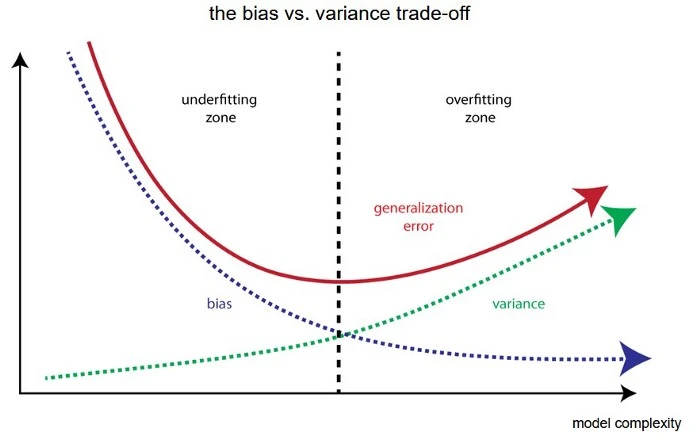## Category Archives: Machine Learning### Relationship between bias, variance, and test set MSE

In all three cases, the variance increases and the bias decreases as the method’s flexibility increases. However, the flexibility level corresponding to the optimal test MSE differs considerably among the three data sets, because the squared bias and variance change at different rates in each of the data sets.

### Difference Between Classification and Regression in Machine Learning

Classification vs Regression Classification predictive modeling problems are different from regression predictive modeling problems. Classification is the task of predicting a discrete class label. Regression is the task of predicting a continuous quantity.

### What are “ensemble methods”?

An ensemble methods are technique which uses multiple independent similar or different models and then combine them to produce improved results. Ensemble methods usually produces more accurate solutions than a single model would. The motivation for using ensemble models is to reduce bias or variance. For e.g. A random forest is an ensemble of multiple decision […]

### Entropy, Information gain, and Gini Index: Decision Tree

The decision tree algorithm is one of the widely used methods for inductive inference. It approximates discrete-valued target functions while being robust to noisy data and learns complex patterns in the data. The family of decision tree learning algorithms includes algorithms like ID3, CART, ASSISTANT, etc. They are supervised learning algorithms used for both, classification […]### What is bias and variance in machine learning?

Some models are too simplistic and ignore important relationships in the training data, which could have improved their predictions. Such models are said to have high bias. When a model has high bias, its predictions are consistently off, at least for certain regions of the data if not the whole range. For example, if you try […]